##### Properties of Graphs

Graph theory is the study of properties or invariants of graphs. Among the properties of interest are such things as connectivity, cycle structure, and chromatic number. Here, we demonstrate how to compute several different graph invariants.

An undirected graph is connected if there exists a path between any pair of vertices. Deleting an edge from a connected graph can disconnect it. Such an edge is called a bridge.

`ConnectedQ[ DeleteEdge[ Star, {1,10} ] ]`
`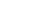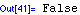`

GraphUnion can be used to create disconnected graphs.

`ConnectedComponents[ GraphUnion[CompleteGraph, CompleteGraph] ]`
`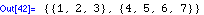`

An orientation of an undirected graph $G$ is an assignment of exactly one direction to each of the edges of $G$. This orientation of a wheel directs each edge in the outer cycle in the same direction, and completes it by giving the center an inŁdegree of $n - 1$ and outŁdegree of 1.

`ShowGraph[ OrientGraph[Wheel], Directed];`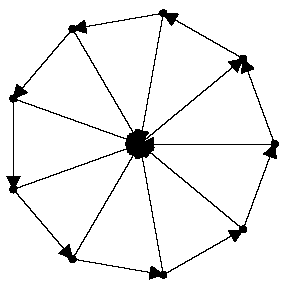An articulation vertex of a graph $G$ is a vertex whose deletion disconnects $G$. Any graph with no articulation vertices is said to be biconnected. A graph with a vertex of degree 1 cannot be biconnected, since deleting the other vertex that defines its only edge disconnects the graph.

`BiconnectedComponents[ RealizeDegreeSequence[{4,4,3,3,3,2,1}] ]`
``

The only articulation vertex of a star is its center, even though its deletion leaves $n - 1$ connected components. Deleting a leaf leaves a connected tree.

`ArticulationVertices[ Star ]`
`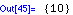`

Every edge in a tree is a bridge.

`Bridges[ RandomTree ]`
`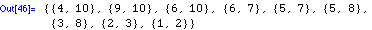`

A graph is said to be $k$Łconnected if there does not exist a set of $k - 1$ vertices whose removal disconnects the graph. The wheel is the basic triconnected graph.

`VertexConnectivity[Wheel]`
`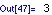`

A graph is $k$ŁedgeŁconnected if there does not exist a set of $k - 1$ edges whose removal disconnects the graph. The edge connectivity of a graph is at most the minimum degree $\delta$, since deleting those edges disconnects the graph. Complete bipartite graphs realize this bound.

`EdgeConnectivity[CompleteGraph[3,4]]`
`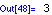`

These two complete bipartite graphs are isomorphic, since the order of the two stages is simply reversed. Here, all isomorphisms are returned.

`Isomorphism[CompleteGraph[3,2], CompleteGraph[2,3], All]`
``

A graph is selfŁcomplementary if it is isomorphic to its complement. The smallest nonŁtrivial selfŁcomplementary graphs are the path on four vertices and the cycle on five.

`SelfComplementaryQ[ Cycle ] && SelfComplementaryQ[ Path ]`
`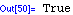`

A directed graph with half the edges is almost certain to contain a cycle. Directed acyclic graphs are often called DAGs.

`AcyclicQ[ RandomGraph[7,0.5,Directed], Directed]`
`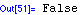`

The girth of a graph is the length of its shortest cycle. The girth of a complete graph is 3, since it contains a triangle, the smallest possible cycle.

`Girth[ CompleteGraph ]`
`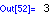`

An Eulerian cycle is a complete tour of all the edges of a graph. An Eulerian cycle of a bipartite graph bounces back and forth between the stages.

`EulerianCycle[ CompleteGraph[4,4] ]`
`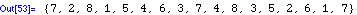`

A Hamiltonian cycle of a graph $G$ is a cycle that visits every vertex in $G$ exactly once, as opposed to an Eulerian cycle, which visits each edge exactly once. $K_\left\{n,n\right\}$ for $n > 1$ are the only Hamiltonian complete bipartite graphs.

`HamiltonianCycle[CompleteGraph[3,3], All]`
`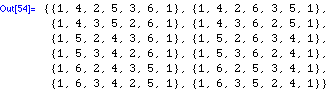`

The divisibility relation between integers is reflexive since each integer divides itself and antiŁsymmetric, since $x$ cannot divide $y$ if $x > y$. Finally, it is transitive, as $x \ y$ implies $y = c x$ for some integer $c$, so $y \ z$ implies $x \ z$.

`ShowLabeledGraph[ g = MakeGraph[Range,(Mod[#1,#2]==0)&] ];`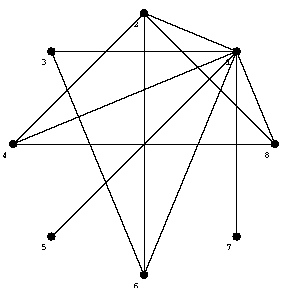Since the divisibility relation is reflexive, transitive, and antiŁsymmetric, it is a partial order.

`PartialOrderQ[g]`
`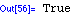`

A graph $G$ is transitive if any three vertices $x, y, z$ such that edges $\left\{x, y\right\}, \left\{y, z\right\} \in G$ imply $\left\{x, z\right\} \in G$. The transitive reduction of a graph $G$ is the smallest graph $R\left(G\right)$ such that $C\left(G\right) = C\left(R\left(G\right)\right)$. The transitive reduction eliminates all implied edges in the divisibility relation, such as $4 \ 8$, $1 \ 4$, $1 \ 6$, and $1 \ 8$.

`ShowLabeledGraph[TransitiveReduction[g]]`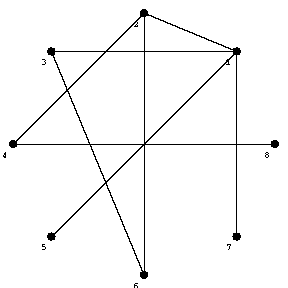`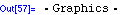`

The Hasse diagram clearly shows the lattice structure of the Boolean algebra, the partial order defined by inclusion on the set of subsets.

`ShowLabeledGraph[  HasseDiagram[MakeGraph[Subsets,  ((Intersection[#2,#1]===#1)&&(#1 != #2))&]],  Subsets ];`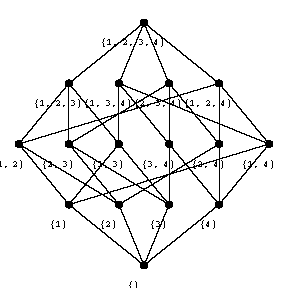A topological sort is a permutation $p$ of the vertices of a graph such that an edge $\left\{i, j\right\}$ implies $i$ appears before $j$ in $p$. A complete directed acyclic graph defines a total order, so there is only one possible output from TopologicalSort.

`TopologicalSort[ MakeGraph[Range,(#1 > #2)&] ]`
`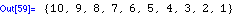`

Any labeled graph $G$ can be colored in a certain number of ways with exactly $k$ colors $1, ..., k$. This number is determined by the chromatic polynomial of the graph.

`ChromaticPolynomial[ GraphUnion[CompleteGraph[2,2], Cycle], z ]`
``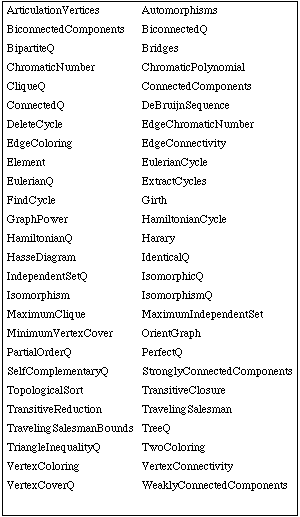Combinatorica functions for properties of graphs.